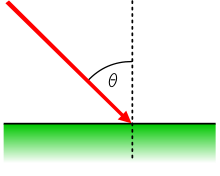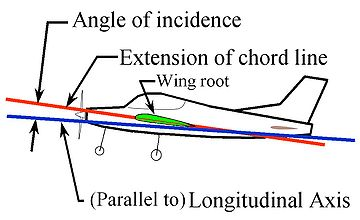# Angle of incidence

﻿
Angle of incidenceAngle of incidence

Angle of incidence is a measure of deviation of something from "straight on", for example:

• in the approach of a ray to a surface, or
• the angle at which the wing or horizontal tail of an airplane is installed on the fuselage, measured relative to the axis of the fuselage.

## Optics

In geometric optics, the angle of incidence is the angle between a ray incident on a surface and the line perpendicular to the surface at the point of incidence, called the normal. The ray can be formed by any wave: optical, acoustic, microwave, X-ray and so on. In the figure above, the red line representing a ray makes an angle θ with the normal (dotted line). The angle of incidence at which light is first totally internally reflected is known as the critical angle. The angle of reflection and angle of refraction are other angles related to beams.

### Grazing angle

When dealing with a beam that is nearly parallel to a surface, it is sometimes more useful to refer to the angle between the beam and the surface, rather than that between the beam and the surface normal, in other words 90° minus the angle of incidence. This angle is called a glancing angle or grazing angle. Incidence at small grazing angle is called "grazing incidence".

Grazing incidence diffraction is used in X-ray spectroscopy and atom optics, where significant reflection can be achieved only at small values of the grazing angle. Ridged mirrors are designed for reflection of atoms coming at small grazing angle. This angle is usually measured in milliradians.

Determining the grazing angle with respect to a planar surface is trivial, but the computation for almost any other surface is significantly more difficult. The exact solution for a sphere (which has important applications in astronomy and computer graphics) was an open problem for nearly 50 years until a closed-form result was derived by mathematicians Allen R Miller and Emanuel Vegh in 1991.

## Angle of incidence of fixed-wing aircraftAngle of incidence of an airplane wing on an airplane.

On fixed-wing aircraft, angle of incidence is the angle between the chord line of the wing where the wing is mounted to the fuselage and the longitudinal axis of the fuselage. The angle of incidence is fixed in the design of the aircraft by the mounting of the wing to the fuselage.

The term can also be applied to horizontal surfaces in general (such as canards or horizontal stabilizers) for the angle they make relative the longitudinal axis of the fuselage.

The figure to the right shows a side view of an airplane. The extended chord line of the wing root (red line) makes an angle with the longitudinal axis (roll axis) of the aircraft (blue line). Wings are typically mounted at a small positive angle of incidence, to allow the fuselage to be "flat" to the airflow in normal cruising flight. Angles of incidence of about 6° are common on most general aviation designs.

Other terms for angle of incidence in this context are rigging angle and rigger's angle of incidence. It should not be confused with the angle of attack, which is the angle the wing chord presents to the airflow in flight. Note that some ambiguity in this terminology exists, as some engineering texts that focus solely on the study of airfoils and their medium may use either term when referring to angle of attack. The use of the term "angle of incidence" to refer to the angle of attack occurs chiefly in British usage.

Wikimedia Foundation. 2010.

### Look at other dictionaries:

• Angle of incidence — Incidence In ci*dence, n. [Cf. F. incidence.] [1913 Webster] 1. A falling on or upon; an incident; an event; an occurrence. [Obs.] Bp. Hall. [1913 Webster] 2. (Physics) The direction in which a body, or a ray of light or heat, falls on any… …   The Collaborative International Dictionary of English

• Angle D'incidence — Incidence (optique) Pour les articles homonymes, voir incidence. Θ est l angle d incidence L angle d incidence est l angle entre la d …   Wikipédia en Français

• Angle of incidence — (A[ e]ronautics) The angle between the chord of an a[ e]rocurve and the relative direction of the undisturbed air current. [Webster 1913 Suppl.] …   The Collaborative International Dictionary of English

• angle of incidence — n the angle that a line (as a ray of light) falling on a surface or interface makes with the normal drawn at the point of incidence * * * the angle made with the perpendicular by a ray of light which strikes a denser or a rarer medium; see… …   Medical dictionary

• angle of incidence — n. 1. the angle that a light ray or electromagnetic wave striking a surface makes with a line perpendicular to the reflecting surface 2. Chiefly Brit. ANGLE OF ATTACK 3. Aeron. a permanent angle between the chord of an airfoil and the… …   English World dictionary

• Angle d'incidence — ● Angle d incidence angle formé par la corde d un profil d aile et l axe longitudinal de l avion …   Encyclopédie Universelle

• angle of incidence — i. The acute angle formed between the chord line of an airfoil and the longitudinal axis of the aircraft on which it is mounted. This is the angle at which the airfoil is attached to an aircraft fuselage when the aircraft is in rigging position.… …   Aviation dictionary

• angle of incidence —    The angle of incidence as used here conforms to that used in optics to describe reflection and refraction of light rays. The angle is measured with respect to the normal to the surface, rather than to the surface itself. The normal is an… …   Forensic science glossary

• Angle of Incidence —   In reference to solar energy systems, the angle at which direct sunlight strikes a surface; the angle between the direction of the sun and the perpindicular to the surface. Sunlight with an incident angle of 90 degrees tends to be absorbed,… …   Energy terms

• angle d’incidence — kritimo kampas statusas T sritis fizika atitikmenys: angl. incidence angle vok. Einfallswinkel, m; Einfallwinkel, m rus. угол падения, m pranc. angle d’incidence, m …   Fizikos terminų žodynas